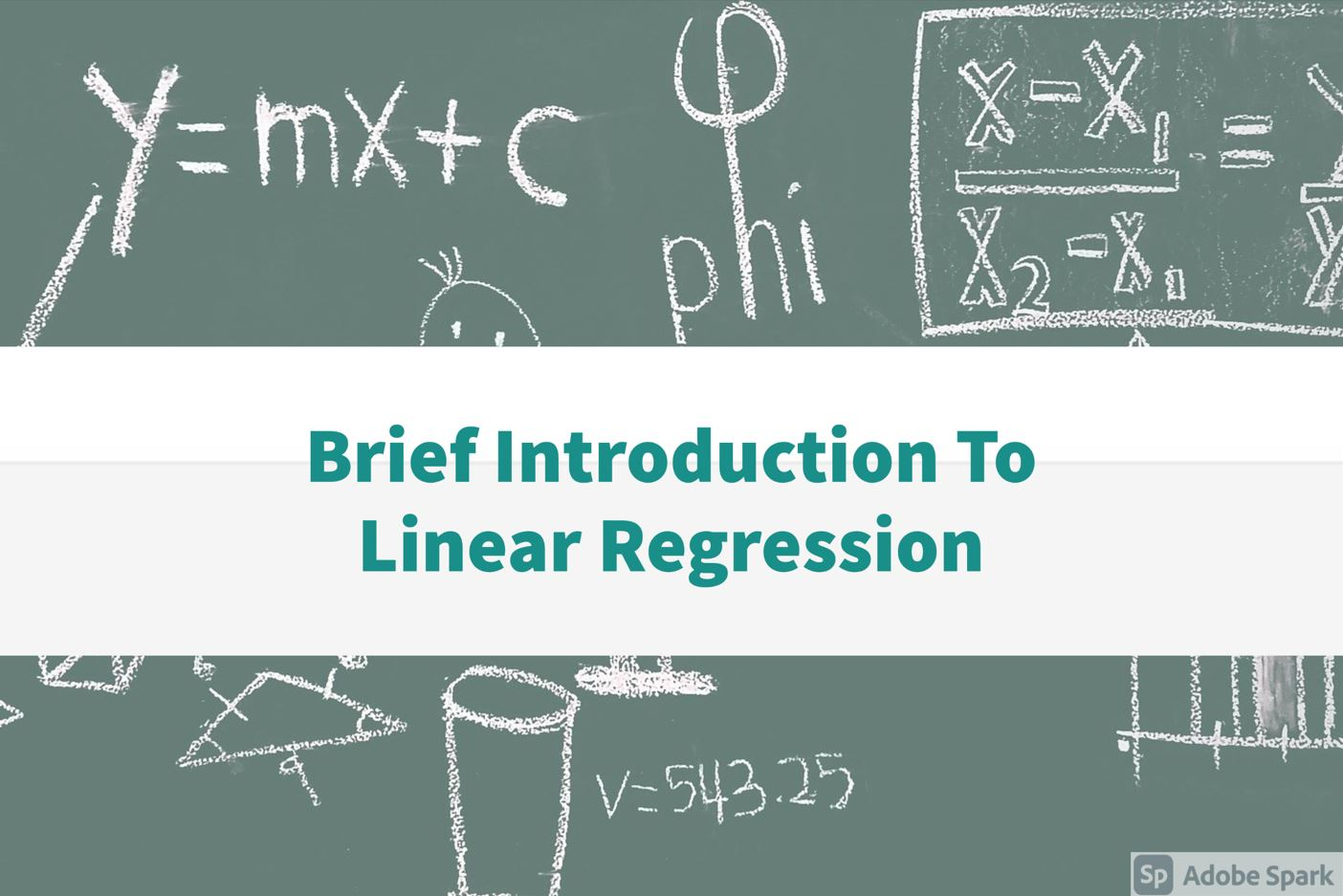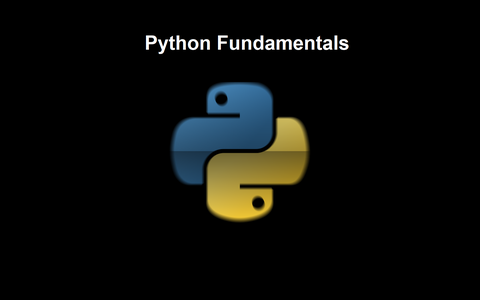All

# Logical Conditions

Python supports the usual logical conditions from mathematics:

• Equals: a == b
• Not Equals: a != b
• Less than: a < b
• Less than or equal to: a <= b
• Greater than: a > b
• Greater than or equal to: a >= b

These conditions are used in many ways, in loops or in calling methods/functions or as a restriction on data.

In python indentations are used instead of curly braces

It means if we want to include some lines inside an if block so we will give 4 spaces (conventionally) or 1 tab instead of encolsing whole block in {} , Same thing goes for functions and classes

# Indentation

To understand Indentation, lets compare python with C so

this python code

```if a<b:
#Do something
#Do something
#Do something
#Do something - if block ends here
#This line is out side of if block
```

is similar to this piece of script in C.

```if (a<b)
{
//Do something
//Do something
//Do something
//Do something - if block ends here
}

//This line is out side of if block
```

# Examples

```a = 2
b = 4
if a<b:
print("a is less than b")
elif a>b:
print("a is less than b")
elif a==b:
print("a is equal to b")
else:
print("Do something else")
```

### Subscribe to my youtube channel

AllBest Programming laptops to buy in 2021

May 23, 2021, 12:19 p.m.#### Start watching python tutorialsPython Essentials

April 21, 2021, 6:08 p.m.